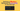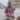# Solving a Mystery Behavior of parseInt() in JavaScript

Posted April 20, 2021Start discussion

`parseInt()` is a built-in JavaScript function that parses integers from numerical strings. For example, let’s parse the integer from the numeric string `'100'`:

``````const number = parseInt('100');
number; // 100``````

As expected, `'100'` is parsed to integer `100`.

`parseInt(numericalString, radix)` also accepts a second argument: the radix at which the numerical string argument is. The radix argument allows you to parse integers from different numerical bases, the most common being 2, 8, 10, and 16.

Let’s use `parseInt()` to parse a numerical string in base 2:

``````const number = parseInt('100', 2);
number; // 4``````

`parseInt('100', 2)` parses `'100'` as an integer in numerical base 2: thus it returns the value `4` (in decimal).

That’s pretty much a short introduction to `parseInt()`.

## 1. A mystery behavior of parseInt()

`parseInt(numericalString)` always converts its first argument to a string (if it’s not a string), then parses that numeric string to the integer value.

That’s why you can (but should’t!) use `parseInt()` to extract the integer part of float numbers:

``````parseInt(0.5);      // => 0
parseInt(0.05);     // => 0
parseInt(0.005);    // => 0
parseInt(0.0005);   // => 0
parseInt(0.00005);  // => 0
parseInt(0.000005); // => 0``````

Try the demo.

Extracting the integer part of floats like `0.5`, `0.05`, etc. results in `0`. This works as expected.

What about extracting the integer part of `0.0000005`?

``parseInt(0.0000005); // => 5``

Try the demo.

`parseInt()` parses the float `0.0000005` to… `5`. Interesting and kind of unexpected…

Why does `parseInt(0.0000005)` have such a mystery behavior?

## 2. Solving the mystery of parseInt()

Let’s look again at what `parseInt(numericalString)` does with its first argument: if it’s not a string, then it is converted to a string, then parsed, and the parsed integer returned.

That might be the first clue.

Let’s try then to convert manually the floats to a string represenation:

``````String(0.5);      // => '0.5'
String(0.05);     // => '0.05'
String(0.005);    // => '0.005'
String(0.0005);   // => '0.0005'
String(0.00005);  // => '0.00005'
String(0.000005); // => '0.000005'

String(0.0000005); // => '5e-7'``````

Try the demo.

The explicit conversion to a string of `String(0.0000005)` behaves differently than other floats: it’s a string representation of the exponential notation!

That’s the second — and a significant clue!

And when the expontential notiation is parsed to an integer, you get the number `5`:

``````parseInt(0.0000005); // => 5
// same as
parseInt(5e-7);      // => 5
// same as
parseInt('5e-7');    // => 5``````

Try the demo.

`parseInt('5e-7')` takes into consideration the first digit `'5'`, but skips `'e-7'`.

Mystery solved! Because `parseInt()` always converts its first argument to a string, the floats smaller than 10-6 are written in an exponential notation. Then `parseInt()` extracts the integer from the exponential notation of the float.

On a side note, to safely extract the integer part of a float number I recommend `Math.floor()` function:

``````Math.floor(0.5);      // => 0
Math.floor(0.05);     // => 0
Math.floor(0.005);    // => 0
Math.floor(0.0005);   // => 0
Math.floor(0.00005);  // => 0
Math.floor(0.000005); // => 0

Math.floor(0.0000005); // => 0``````

Try the demo.

## 3. Conclusion

`parseInt()` is the function that parses numerical strings to integers.

Care must be taken when trying to extract the integer part of floats using `parseInt()`.

Floats smaller than 10-6 (e.g. `0.0000005` which is same as 5*10-7) conversed to a string are written in the exponential notation (e.g. `5e-7` is the exponential notation of `0.0000005`). That’s why using such small floats with `parseInt()` leads to unexpected results: only the significat part (e.g. `5` of `5e-7`) of the exponential notiation is parsed.

Side challenge: can you explain why `parseInt(999999999999999999999)` equals `1`? Write your considerations in a comment below!

## Quality posts into your inbox

I regularly publish posts containing:

• Important JavaScript concepts explained in simple words
• Overview of new JavaScript features
• How to use TypeScript and typing
• Software design and good coding practices